# Surface Areas and Volumes Class 10 Formulas PDF

## Surface Areas and Volumes Class 10 Formulas PDF Summary

Dear readers, here we are offering Surface Areas and Volumes Class 10 Formulas PDF to all of you. Surface area and volume are calculated for any three-dimensional geometrical shape. The surface area of any given object is the area or region occupied by the surface of the object. Whereas volume is the amount of space available in an object.
We may see a variety of solid shapes all around us. Using specific formulas, we may calculate the surface area and volume of various solid shapes. A cube, cuboids, cylinders, cones, and other solid bodies can be used. We will go over how to calculate the surface area and volume for each of these shapes.

### Surface Areas and Volumes Class 10 Formulas PDF

Below given is the table for the calculating Surface area and Volume for the basic geometrical figures:

 Name Perimeter Total Surface Area Curved Surface Area/Lateral Surface Area Volume Figure Square 4b b2 —- —-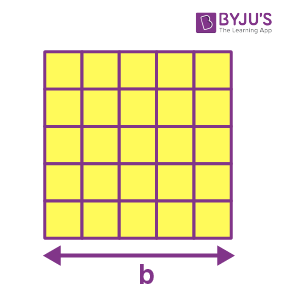Rectangle 2(w+h) w.h —- —-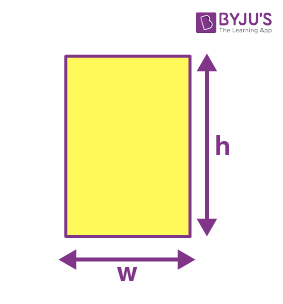Parallelogram 2(a+b) b.h —- —-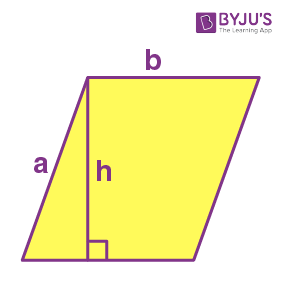Trapezoid a+b+c+d 1/2(a+b).h —- —-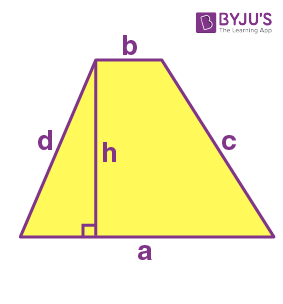Circle 2 π r π r2 —- —-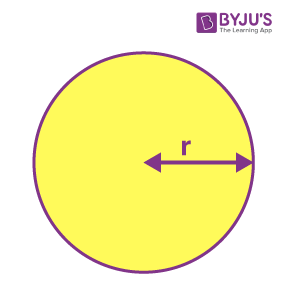Ellipse 2π√(a2 + b2)/2 π a.b —- —-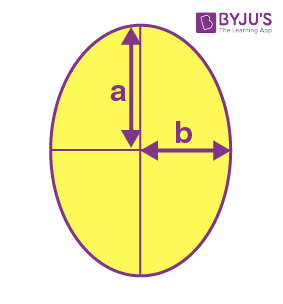Triangle a+b+c 1/2 * b * h —- —-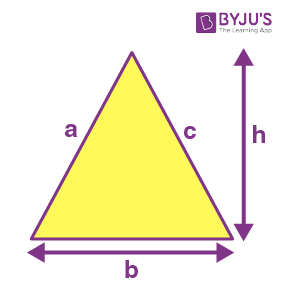Cuboid 4(l+b+h) 2(lb+bh+hl) 2h(l+b) l * b * h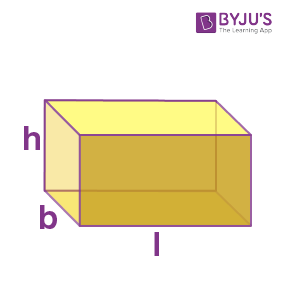Cube 6a 6a2 4a2 a3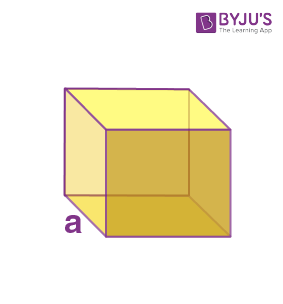Cylinder —- 2 π r(r+h) 2πrh π r2 h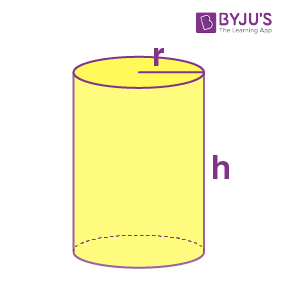Cone —- π r(r+l) π r l 1/3π r2 h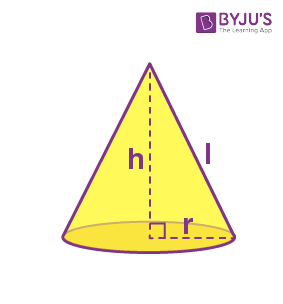Sphere —- 4 π r2 4π r2 4/3π r3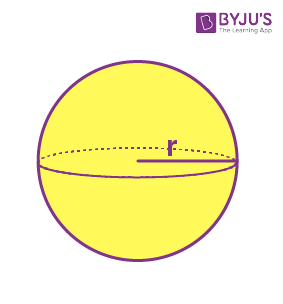Hemisphere —- 3 π r2 2 π r2 2/3π r3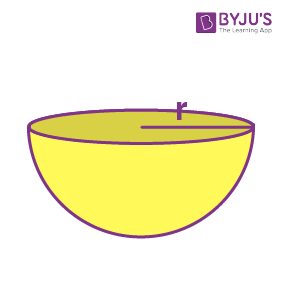### Surface Areas and Volumes Class 10 Formulas – Solved Examples

Q.1. What is the curved surface area of a hemisphere if the diameter is 12cm. 12cm.
Ans: The diameter is 12cm.12cm.
The curved surface area of the hemisphere =2πr2=2×227×6×6cm2=2πr2=2×227×6×6cm2
=226.28cm2=226.28cm2
Q.2. Find the length of the sides of the cube if its volume is 216cm3.216cm3.
Ans: Given, the volume of the cube =216cm3=216cm3
Let the length of the sides is a.a.
We know,
The volume of a cube =(side)3=(side)3
Substituting the value we get,
a3=216a3=216
⇒a=216−−−√3⇒a=6cm⇒a=2163⇒a=6cm
Hence, the side of the cube is 6cm.6cm.
Q.3. If the diagonal is 43–√cm.43cm. Find the volume of the cube.
Ans: We know, V=d333√⇒V=(43√)333√=64×33√33√⇒V=64cm3V=d333⇒V=(43)333=64×3333⇒V=64cm3
Hence, the volume of the cube is 64cm3.64cm3.
Q.4. The slant height of a frustum of a cone is 4cm,4cm, and the perimeters (circumference) of its circular ends are 18cm18cm and 6cm.6cm. Find the curved surface area of the frustum.
Ans: Given, l=4cml=4cm
Circumference of a circular end =18cm=18cm
⇒2πr1=18cm⇒2πr1=18cm
⇒πr1=9cm⇒πr1=9cm
Circumference of another circular end =6cm=6cm
⇒2πr2=6cm⇒2πr2=6cm
⇒πr2=3cm⇒πr2=3cm
We know that curved surface area =πl(r1+r2)=l(πr1+πr2)=πl(r1+r2)=l(πr1+πr2)
=4×(9+3)cm2=4×(9+3)cm2
=48cm2=48cm2
Hence, the curved surface area of the frustum is 48cm2.48cm2.
Q.5. Madhu prepares a birthday cap with a piece of paper in the form of a right circular cone of radius 33 inches and a height of 44 inches. Find the slant height of the birthday cap made by her.
Ans: Given that, the birthday cap prepared by Madhu is in the shape of a right circular cone.
The radius of the cone (r)=3(r)=3 inches and the height of the cone (h)=4(h)=4 inches.
It is known that the slant height of the cone is given by l=r2+h2−−−−−−√.l=r2+h2.
⇒l=32+42−−−−−−√⇒l=32+42
⇒l=9+16−−−−−√⇒l=9+16
⇒l=25−−√=5⇒l=25=5 inches
Hence, the slant height of the birthday cap is 55 inches.